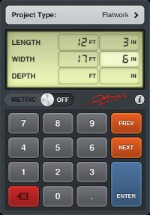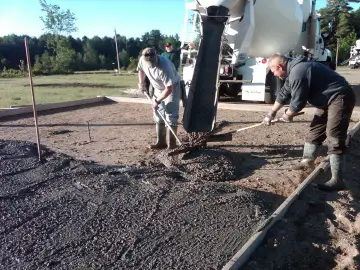# Calculating ConcreteCalculating concrete for your project is easy with this free concrete calculator download for your desktop computer.  All you have to do is enter the dimensions and thickness, hit calculate, and it figures the concrete yardage for you.

If you know the cost per yard, you can enter that in as well and the calculator will estimate the total concrete cost. This calculator will estimate concrete for floors, slabs, footings, cylinders and sono-tubes.

At the bottom of this page is a formula to calculate concrete for flatwork.  It's good to know in case your laptop or phone isn't handy.

There is also an APP for the Iphone or Android phone you can download that is great for calculating concrete yardage.  ConstructionBuddy.com has a concrete calculator for each type of phone. It cost .99 cents but is well worth it.

I personally use the one for the Android a lot and haven't had any problems.  This APP will calculate concrete for slabs, blocks, footings and sono-tubes. It also does conversions and will switch between metric and standard units. Very handy!

Figuring how much concrete to order for a slab like the one below takes about 20 seconds with this APP for your smartphone.## HOW TO CALCULATE CONCRETE

Another way you can calculate the amount of concrete you need is to use this formula: L x W x H /27  -- This formula will figure cubic yardage for a slab or floor.  Here's an example:

Let's calculate the concrete for a 20 foot x 20 foot x 6 inch thick concrete slab.

1. 20 x 20 = 400
2. 400 x .5 = 200  ( The thickness or Height of the slab is 6 inches or .5 feet), (4 inches would be .33 feet)
3. 200 / 27 = 7.4 cubic yards

I always add a little extra, 1/4 - 1/2 yard, if I'm ordering the concrete from a redi-mix company, you never want to run out.

One more way to share everything-about-concrete.com